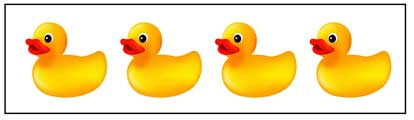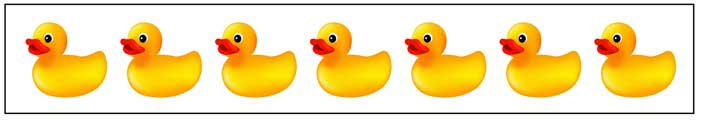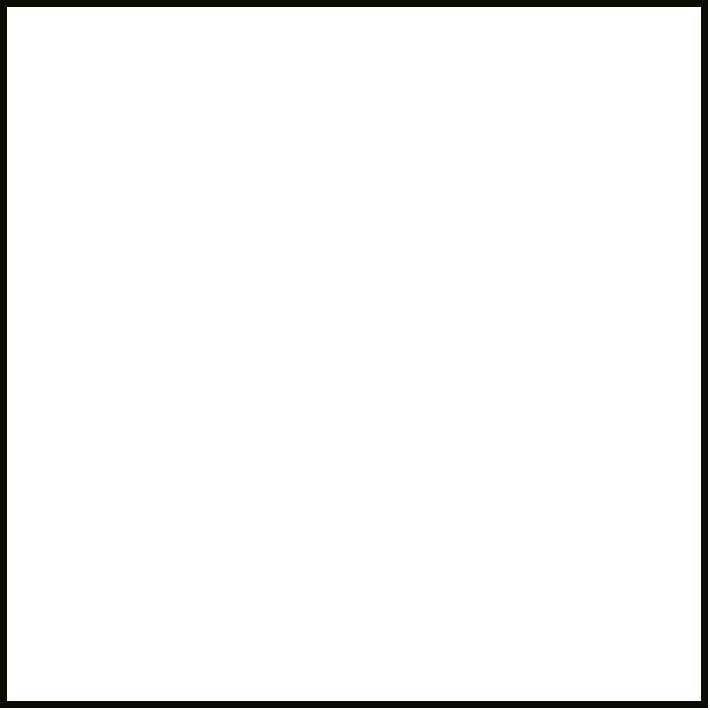Tuesday, September 28, 2021# Rubber ducks (m3.oa.1) math practice

-

This test question, released by the Partnership for the Assessment of Readiness for College and Career (PARCC), is discussed here in the interest of helping third-grade students, their parents, and friends understand more clearly the knowledge and skills that third graders should have in math by the end of the school year (2018 #1).### Drag and drop the ducks into the box.ModelFor this problem, you have to select the pieces and drag them into the box labeled “Model” to make a model of the multiplication expression “4 × 7.” This blog doesn’t let me make them drag-and-drop pieces, but on a test, that’s how you would answer this question.

• 4 pieces with 7 ducks each, or
• 7 pieces with 4 ducks each

Having four pieces with 7 ducks each is like saying 7 + 7 + 7 + 7. That equals 28, and it is just another way of saying 4 × 7. So the little model with the ducks is exactly what the question asked for.

On the other hand, with multiplication, it doesn’t matter what order you write the two numbers in. That means that 4 × 7, as the problem asks for, is exactly the same as 7 × 4. And that means it’s just as correct to have seven pieces with 4 ducks each in the box. That’s like saying 4 + 4 + 4 + 4 + 4 + 4 + 4, or seven groups with 4 ducks each. That also equals 28.

Using a model like this, you can see that 4 × 7 is the same as 7 × 4. You get the same number, which is 28 in this case. From this, you can figure out that it doesn’t matter which way you write the numbers in a multiplication problem, because you get the same answer, regardless of the order.

If You Don't Understand This Problem
You can think of multiplication as addition that gets repeated a certain number of “times.” For example, 3 × 4, which you say as “three times four,” means the same thing as adding 3 to itself 4 times. 3 + 3 + 3 + 3 = 12, so 3 × 4 = 12.

• When you create a model for multiplication, you have to include groups with one number of objects in it. How many groups should you include? Whatever the second number is in the multiplication problem.

If you were able to see the ducks in the box on this blog, using seven of the picture with 4 ducks each would make seven “rows” of four ducks each. Using four of the picture with 7 ducks each would make seven “columns” of four ducks each. Whether you put the 4 in the columns and the 7 in the rows or put the 7 in the columns and the 4 in the rows, you still get 28 ducks. Therefore 7 × 4 is the same as 4 × 7, and it equals 28.You can learn more with this type of question by practicing different multiplication problems. For example, make paper models with 2, 3, 5, 6, 8, and 9 ducks each, and drag them into a box on your table to create models that show:

• 5 × 7
• 4 × 8
• 3 × 9

Make sure you line them up in columns and rows so you can see the rectangular arrangement of a model for a multiplication problem.

Internet Resources
Here’s a lesson from the Khan Academy that can help you understand multiplication with models of groups of equal size. It also shows different ways to represent multiplication with models.

## Information for Teachers

This problem tests students’ understanding of the Common Core mathematics standard 3.OA.A.1, which says that by the end of third grade, you should have the ability to “interpret products of whole numbers, for example, interpret 5 × 7 as the total number of objects in 5 groups of 7 objects each. For example, describe a context in which a total number of objects can be expressed as 5 × 7.”

For the first six months following the publication of this article, we encourage and welcome comments about extending this lesson or information about how you have taught this lesson in your classrooms. #LearnTogether #VoxitatisPaul Katulahttps://news.schoolsdo.org
Paul Katula is the executive editor of the Voxitatis Research Foundation, which publishes this blog. For more information, see the About page.

### R. Kelly finally convicted of sex trafficking

0
Singer R Kelly was convicted Monday of 8 counts of sex trafficking and 1 count of racketeering. He faces life in prison.